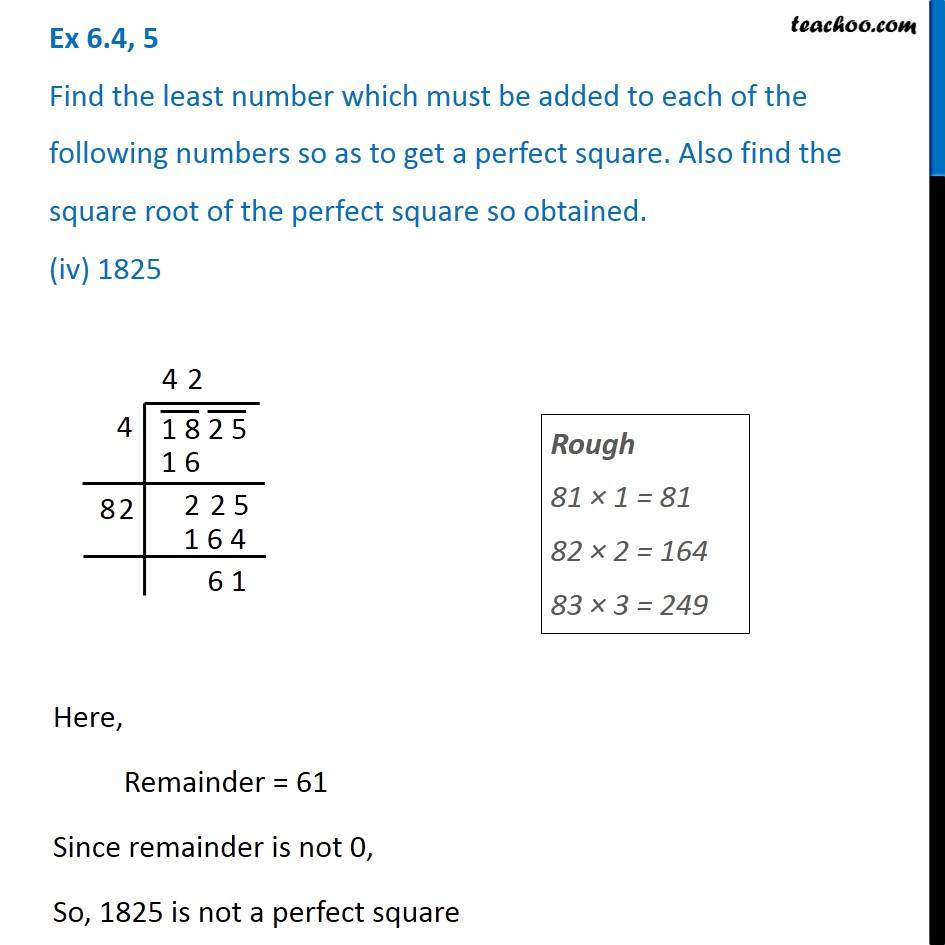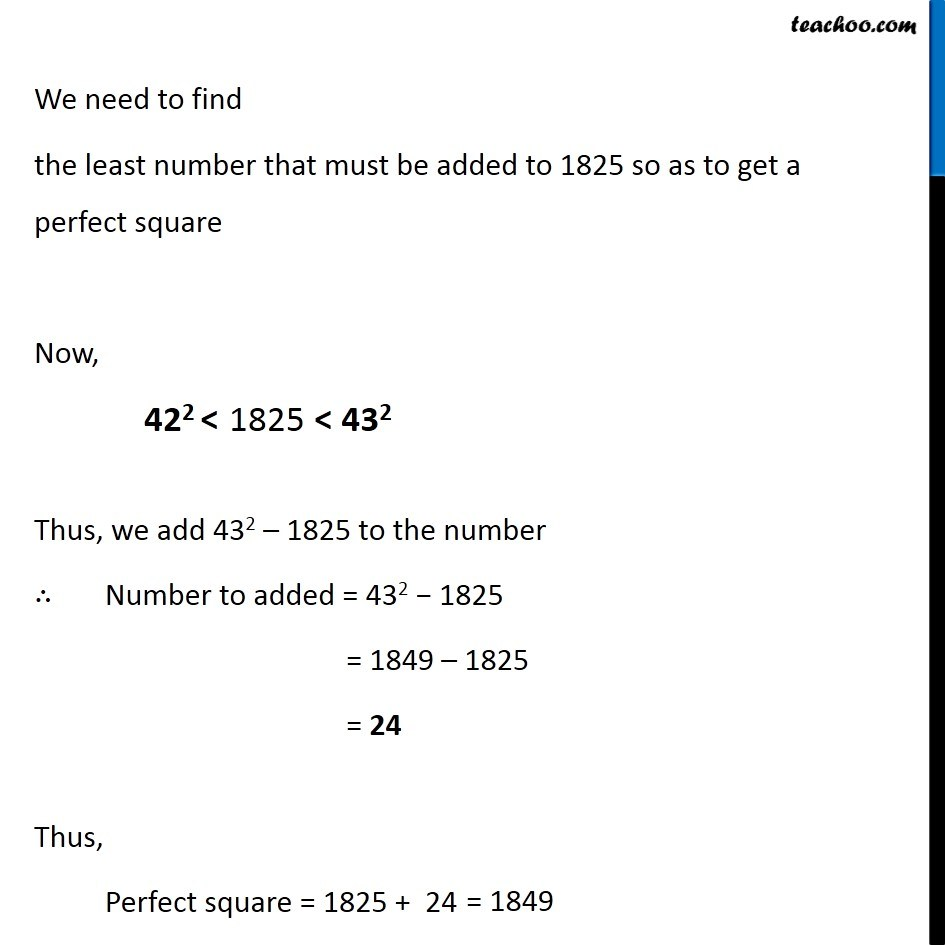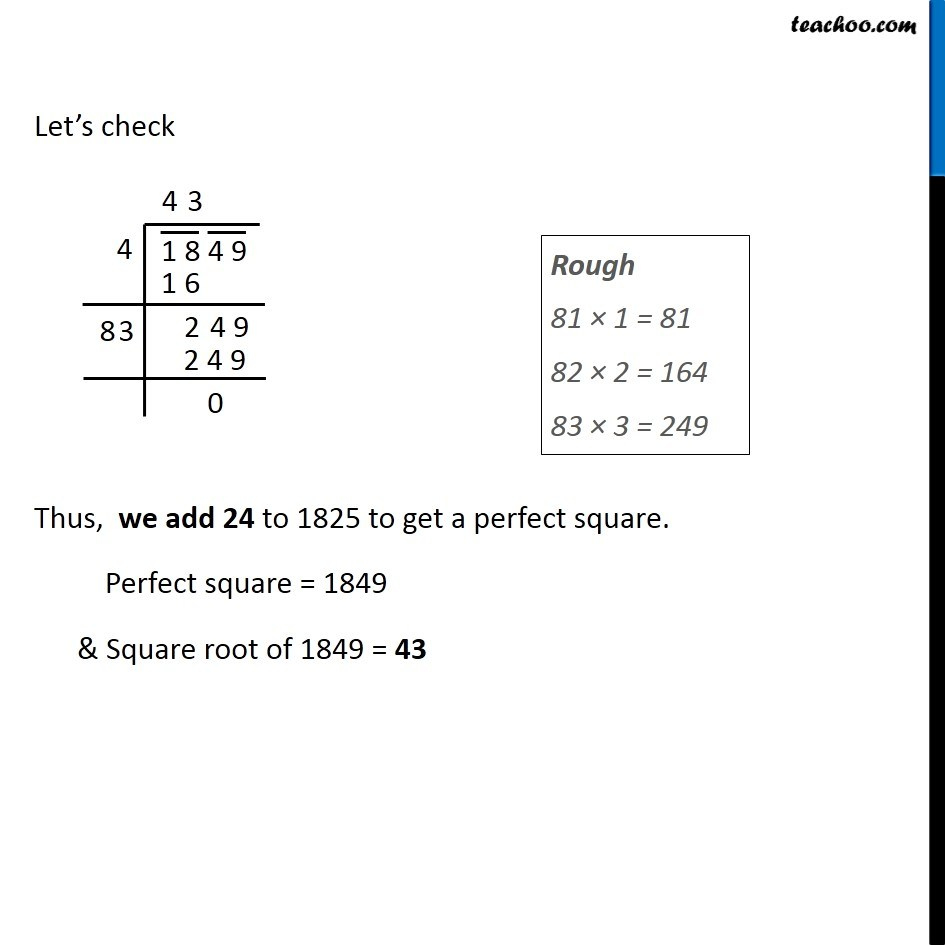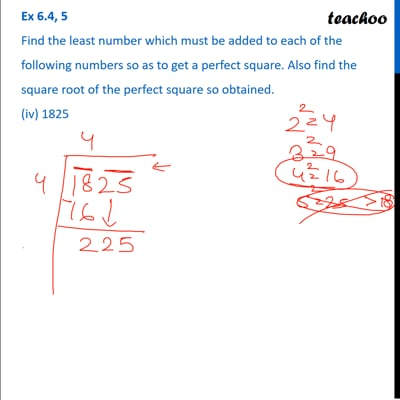Ex 6.4

Chapter 6 Class 8 Squares and Square Roots
Serial order wiseThis video is only available for Teachoo black users

Get live Maths 1-on-1 Classs - Class 6 to 12

### Transcript

Ex 6.4, 5 Find the least number which must be added to each of the following numbers so as to get a perfect square. Also find the square root of the perfect square so obtained. (iv) 1825Rough 81 × 1 = 81 82 × 2 = 164 83 × 3 = 249 Here, Remainder = 61 Since remainder is not 0, So, 1825 is not a perfect square We need to find the least number that must be added to 1825 so as to get a perfect square Now, Thus, we add 432 – 1825 to the number ∴ Number to added = 432 − 1825 = 1849 – 1825 = 24 Thus, Perfect square = 1825 + 24 Let’s check Thus, we add 24 to 1825 to get a perfect square. Perfect square = 1849 & Square root of 1849 = 43 Rough 81 × 1 = 81 82 × 2 = 164 83 × 3 = 249